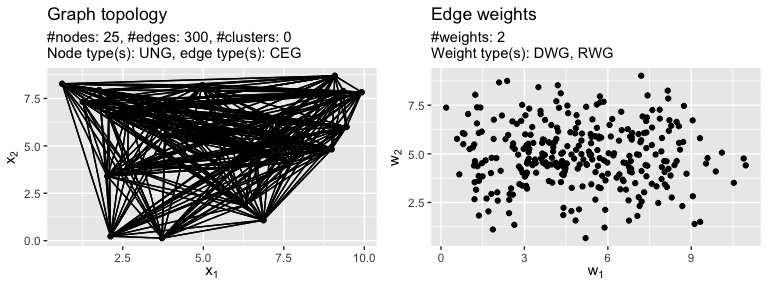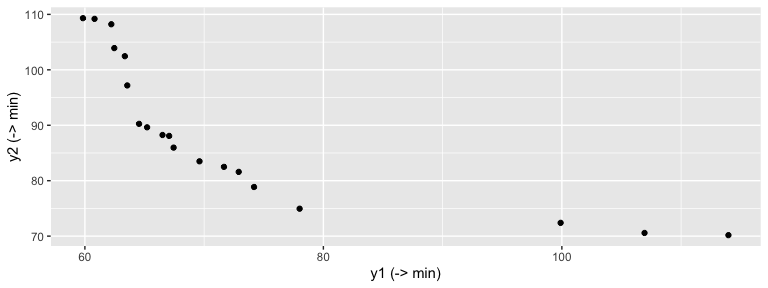# Quickstart

The mcMST package for the statistical programming language R contains methods for solving the multi-criteria minimum spanning tree problem (mcMST).

## Generating a benchmark instance

Here we first generate a bi-criteria graph with n = 25 nodes with the grapherator package. The first weight is the Euclidean distance of node coordinates in [0, 10] x [0, 10] in the Euclidean plane. The second weight follows a normal distribution with mean 5 and standard deviation 1.5. The instance generation process is modular and thus highly flexible (see the grapherator package vignettes for details and further examples).

library(mcMST)
library(grapherator)

set.seed(1) # reproducability
g = graph(0, 10)
g = addWeights(g, generator = addWeightsRandom, method = rnorm, mean = 5, sd = 1.5)
print(g)
#> GRAPHERATOR GRAPH
#> #nodes           : 25 (UNG)
#> #edges           : 300 (CEG)
#> #weights per edge: 2 (DWG,RWG)
do.call(gridExtra::grid.arrange, c(plot(g), list(nrow = 1L)))Plot of the bi-objective sample graph g.

## Running an algorithm

Next, we apply a (30 + 10) genetic algorithm based on the Pruefer-number encoding as proposed by Zhou & Gen to approximate the Pareto-front for max.iter = 500 generations.

res = mcMSTEmoaZhou(g, mu = 30L, lambda = 10L, max.iter = 500L)
head(res$pareto.front, n = 5) #> y1 y2 #> 1 59.83898 109.32049 #> 2 113.96810 70.17428 #> 5 99.89670 72.39588 #> 6 78.00714 74.94721 #> 7 64.54300 90.25433 ecr::plotFront(res$pareto.front)`Approximation of the Pareto-front of the benchmark graph instance.